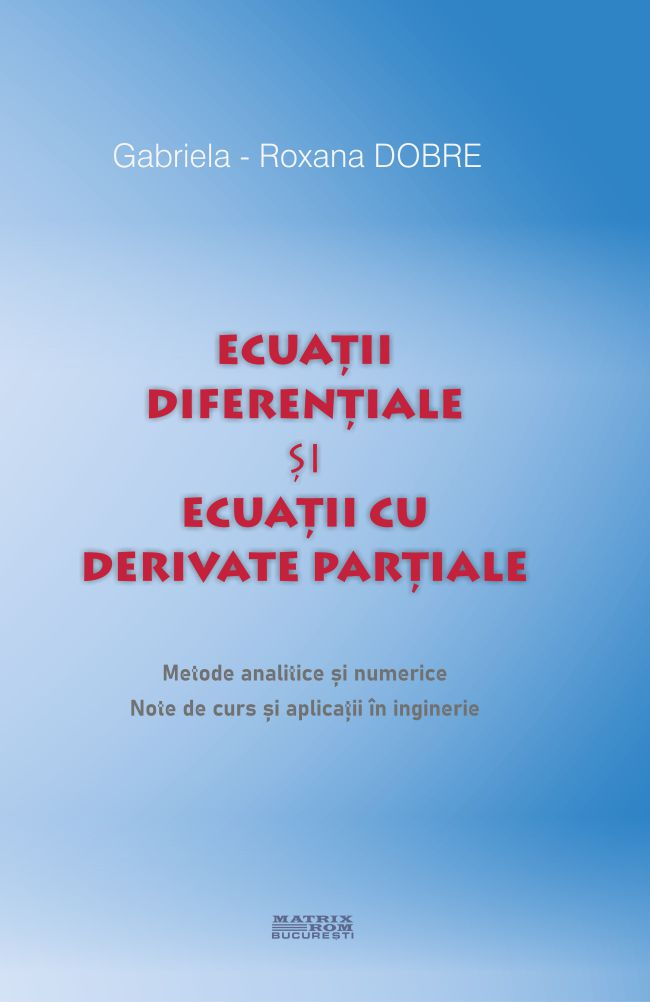Differential equations and equations with partial derivatives. Analytical and numerical methods. Course notes and engineering applications | Matrix Rom
English# Differential equations and equations with partial derivatives. Analytical and numerical methods. Course notes and engineering applications

ISBN: 978-606-25-0574-5
Limba: Romana
Suport: Hartie
66,00 lei
This paper is aimed at students who study mathematical disciplines in universities, engineers or researchers interested in modeling phenomena as well as processes in nature with the help of differential equations and equations with partial derivatives. Often practical problems lead to differential equations or equations with partial derivatives that either cannot be solved using known methods or their solutions have very complicated expressions. In these situations it is preferable to use numerical methods to solve differential equations or equations with partial derivatives. Numerical methods are also part of the fundamental disciplines of training of engineering students.
An important role in the formation of the future specialist in engineering sciences is the adaptation of the curriculum to market requirements. The aim is to provide the basic knowledge on differential and partial lysati equations as well as the analytical and numerical methods of solving them, necessary for specialized courses: physics, mechanics, vibrations, finished element, engineering, elasticity, etc.
For engineers, the purpose of learning the theory of differential equations is to be able to solve practical problems if differential equations are used. Many phenomena and processes in nature are mathematically shaped by differential equations or by equations with partial derivatives. Scientists and engineers need to know how to shape the world in terms of differential equations and equations with partial derivatives, how to solve these equations and how to interpret their solutions. Here are some of their applications in the field of science and engineering: the movement of a material point in a conservative field, the vibrations of an oscillating system, the free fall of bodies, the displacement of an elastic membrane under the action of continuous loading, the propagation of heat in a bar, radioactive disintegration, population growth, various chemical reactions, etc.
The paper tries to be concise and accessible by simplifying exposure without losing mathematical rigor and demonstrates the role of differential equations and equations with partial derivatives in solving typical engineering problems. They are done in four stages:
1) Physical problem
2) Mathematical model: Establishing the differential equation that underlies the problem based on physical principles and geometric properties.
3) Determination of the solution:
- identification of the type of differential equation and the method of resolution
- determination of general and then private solutions (arbitrary constants are determined) using the initial or borderline conditions.
4) Her physical interpretation
The work has a pronounced didactic character organized in nine chapters, in which the theoretical notions presented are accompanied by solved exercises, and each chapter ends with a set of problems proposed for resolution.
The following themes are addressed: "Ordinary differential equations", "Equations with partial derivatives", "Fourier analysis", "Number methods" for solving differential equations and equations with partial derivatives, subjects that are the subject of courses of Special Mathematics or Numerical Methods in technical universities.
Books in eBook format can be viewed through our Bookviewer app.